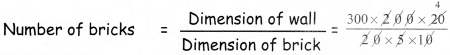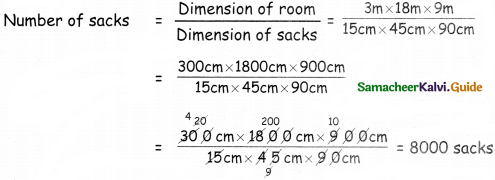Students can download 5th Maths Term 3 Chapter 3 Measurements Ex 3.2 Questions and Answers, Notes, Samacheer Kalvi 5th Maths Guide Pdf helps you to revise the complete Tamilnadu State Board New Syllabus, helps students complete homework assignments and to score high marks in board exams.

## Tamilnadu Samacheer Kalvi 5th Maths Solutions Term 3 Chapter 3 Measurements Ex 3.2

Question 1.
Volume of regular solids such as cube and cuboid can be found by multiplying the dimensions. Complete the given table by finding the volume of the given objects.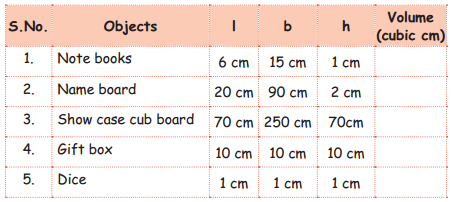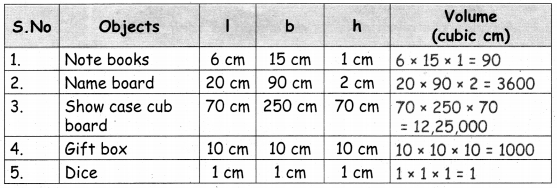Question 2.
Complete the given table by finding the volume of the given objects.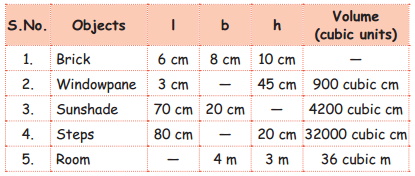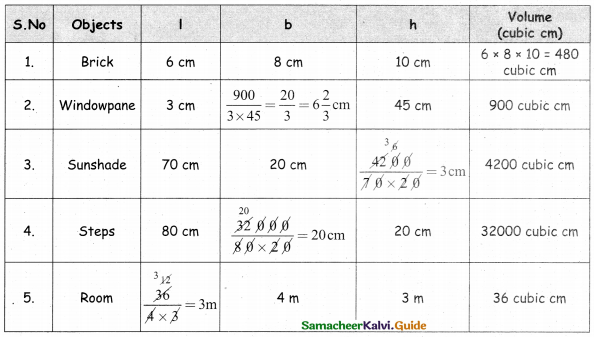Question 3.
Find the number of bricks of dimension 20 cm × 5 cm × 10 cm required to construct a wall of dimension 300 cm × 200 cm × 20 cm.